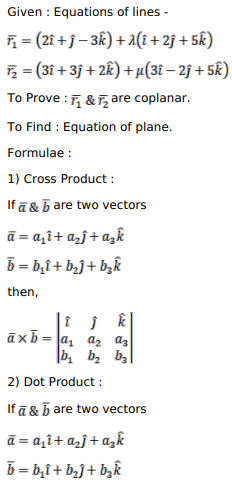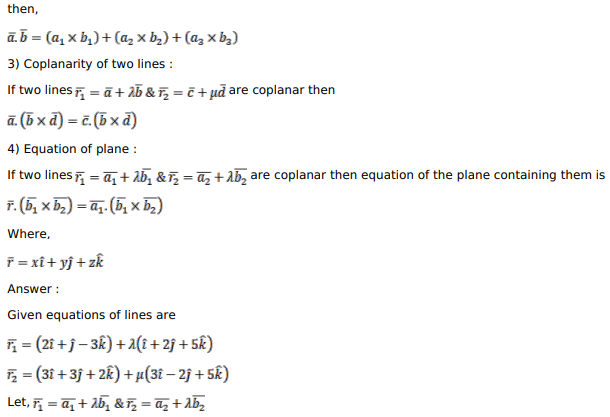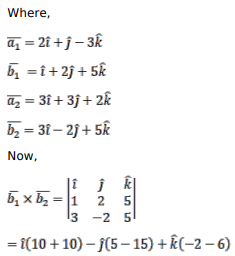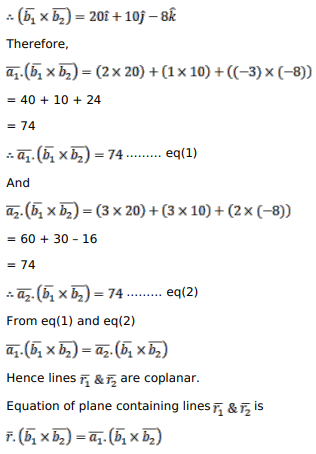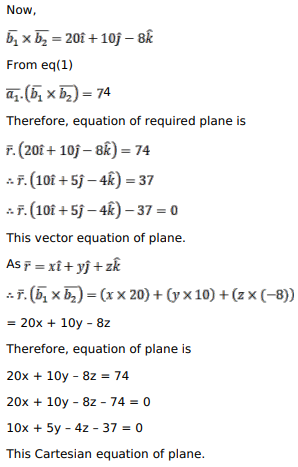# Find the vector and Cartesian equations of a plane containing the two lines

Question:

Find the vector and Cartesian equations of a plane containing the two lines $\bar{r}=(2 \hat{i}+\hat{j}-3 \hat{k})+2(\hat{i}+2 \hat{j}+5 \hat{k})$ and $\bar{r}=(3 \hat{i}+3 \hat{j}+2 \hat{k})+\mu(3 \hat{i}-2 \hat{j}+5 \hat{k})$. Also show that the lines $\bar{r}=(2 \hat{i}+5 \hat{j}+2 \hat{k})+\rho(3 \hat{i}-2 \hat{j}+5 \hat{k})$ lies in the plane.

Solution: